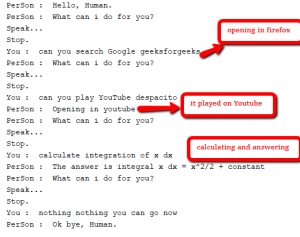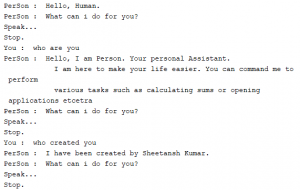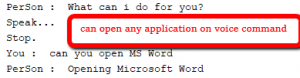Open in App
Not now

# Personal Voice Assistant in Python

• Difficulty Level : Medium
• Last Updated : 25 May, 2022

As we know Python is a suitable language for script writers and developers. Let’s write a script for Personal Voice Assistant using Python. The query for the assistant can be manipulated as per the user’s need. The implemented assistant can open up the application (if it’s installed in the system), search Google, Wikipedia and YouTube about the query, calculate any mathematical question, etc by just giving the voice command. We can process the data as per the need or can add the functionality, depends upon how we code things. We are using Google speech recognition API and google text to speech for voice input and output respectively. Also, for calculating mathematical expression WolframAlpha API can be used. Playsound Package is used to play the saved mp3 sound from the system.

Python external Package Requirements:

-> gTTS – Google Text To Speech, for converting the given text to speech -> speech_recognition – for recognizing the voice command and converting to text -> selenium – for web based work from browser -> wolframalpha – for calculation given by user -> playsound – for playing the saved audio file. -> playaudio – for voice engine in python

Well, let’s get started with code. We will divide each function as a single code for easy understanding. Here’s the main function, with get_audio() and assistant_speaks function. get_audio() function is created to get the audio from user using microphone, the phrase limit is set to 5 seconds (you can change it). Assistant speaks function is created to provide the output according to the processed data.

## Python3

 `# importing speech recognition package from google api` `import` `speech_recognition as sr ` `import` `playsound ``# to play saved mp3 file` `from` `gtts ``import` `gTTS ``# google text to speech` `import` `os ``# to save/open files` `import` `wolframalpha ``# to calculate strings into formula` `from` `selenium ``import` `webdriver ``# to control browser operations`   `num ``=` `1` `def` `assistant_speaks(output):` `    ``global` `num`   `    ``# num to rename every audio file ` `    ``# with different name to remove ambiguity` `    ``num ``+``=` `1` `    ``print``("PerSon : ", output)`   `    ``toSpeak ``=` `gTTS(text ``=` `output, lang ``=``'en'``, slow ``=` `False``)` `    ``# saving the audio file given by google text to speech` `    ``file` `=` `str``(num)``+``".mp3 ` `    ``toSpeak.save(``file``)` `    `  `    ``# playsound package is used to play the same file.` `    ``playsound.playsound(``file``, ``True``) ` `    ``os.remove(``file``)`       `def` `get_audio():`   `    ``rObject ``=` `sr.Recognizer()` `    ``audio ``=` `''`   `    ``with sr.Microphone() as source:` `        ``print``("Speak...")` `        `  `        ``# recording the audio using speech recognition` `        ``audio ``=` `rObject.listen(source, phrase_time_limit ``=` `5``) ` `    ``print``("Stop.") ``# limit 5 secs`   `    ``try``:`   `        ``text ``=` `rObject.recognize_google(audio, language ``=``'en-US'``)` `        ``print``("You : ", text)` `        ``return` `text`   `    ``except``:`   `        ``assistant_speaks("Could ``not` `understand your audio, PLease ``try` `again !")` `        ``return` `0`     `# Driver Code` `if` `__name__ ``=``=` `"__main__":` `    ``assistant_speaks("What's your name, Human?")` `    ``name ``=``'Human'` `    ``name ``=` `get_audio()` `    ``assistant_speaks("Hello, " ``+` `name ``+` `'.'``)` `    `  `    ``while``(``1``):`   `        ``assistant_speaks("What can i do ``for` `you?")` `        ``text ``=` `get_audio().lower()`   `        ``if` `text ``=``=` `0``:` `            ``continue`   `        ``if` `"exit" ``in` `str``(text) ``or` `"bye" ``in` `str``(text) ``or` `"sleep" ``in` `str``(text):` `            ``assistant_speaks("Ok bye, "``+` `name``+``'.'``)` `            ``break`   `        ``# calling process text to process the query` `        ``process_text(text)`

So, we have got an idea here how we are giving voice to the machine and take input from user. The next step and the main step is how you want to process your input. This is just basic code, there is a lot of other algorithms(NLP) can be used to process the text in a proper manner. We have made it static. Also, Wolframalpha api has been used to calculate the calculations part.

## Python3

 `def` `process_text(``input``):` `    ``try``:` `        ``if` `'search'` `in` `input` `or` `'play'` `in` `input``:` `            ``# a basic web crawler using selenium` `            ``search_web(``input``)` `            ``return`   `        ``elif` `"who are you" ``in` `input` `or` `"define yourself" ``in` `input``:` `            ``speak ``=` `'''Hello, I am Person. Your personal Assistant.` `            ``I am here to make your life easier. You can command me to perform` `            ``various tasks such as calculating sums or opening applications etcetra'''` `            ``assistant_speaks(speak)` `            ``return`   `        ``elif` `"who made you" ``in` `input` `or` `"created you" ``in` `input``:` `            ``speak ``=` `"I have been created by Sheetansh Kumar."` `            ``assistant_speaks(speak)` `            ``return`   `        ``elif` `"geeksforgeeks" ``in` `input``:``# just` `            ``speak ``=` `"""Geeks ``for` `Geeks ``is` `the Best Online Coding Platform ``for` `learning."""` `            ``assistant_speaks(speak)` `            ``return`   `        ``elif` `"calculate" ``in` `input``.lower():` `            `  `            ``# write your wolframalpha app_id here` `            ``app_id ``=` `"WOLFRAMALPHA_APP_ID" ` `            ``client ``=` `wolframalpha.Client(app_id)`   `            ``indx ``=` `input``.lower().split().index(``'calculate'``)` `            ``query ``=` `input``.split()[indx ``+` `1``:]` `            ``res ``=` `client.query(``' '``.join(query))` `            ``answer ``=` `next``(res.results).text` `            ``assistant_speaks("The answer ``is` `" ``+` `answer)` `            ``return`   `        ``elif` `'open'` `in` `input``:` `            `  `            ``# another function to open ` `            ``# different application available` `            ``open_application(``input``.lower()) ` `            ``return`   `        ``else``:`   `            ``assistant_speaks("I can search the web ``for` `you, Do you want to ``continue``?")` `            ``ans ``=` `get_audio()` `            ``if` `'yes'` `in` `str``(ans) ``or` `'yeah'` `in` `str``(ans):` `                ``search_web(``input``)` `            ``else``:` `                ``return` `    ``except` `:`   `        ``assistant_speaks("I don't understand, I can search the web ``for` `you, Do you want to ``continue``?")` `        ``ans ``=` `get_audio()` `        ``if` `'yes'` `in` `str``(ans) ``or` `'yeah'` `in` `str``(ans):` `            ``search_web(``input``)`

Now we have processed the input, it’s time for action! There are two functions included that is search_web and open_application. search_web is just a web crawler which uses selenium package to process. It can search google, wikipedia and can open YouTube. You just have to say include the name and it will open it in the Firefox browser. For other browsers, you need to install a proper browser package in selenium. Here we are using webdriver for Firefox. open_application is just a function uses os package to open the application present in the system.

## Python3

 `def` `search_web(``input``):`   `    ``driver ``=` `webdriver.Firefox()` `    ``driver.implicitly_wait(``1``)` `    ``driver.maximize_window()`   `    ``if` `'youtube'` `in` `input``.lower():`   `        ``assistant_speaks("Opening ``in` `youtube")` `        ``indx ``=` `input``.lower().split().index(``'youtube'``)` `        ``query ``=` `input``.split()[indx ``+` `1``:]` `        ``driver.get("http:``/``/``www.youtube.com``/``results?search_query ``=``" ``+` `'+'``.join(query))` `        ``return`   `    ``elif` `'wikipedia'` `in` `input``.lower():`   `        ``assistant_speaks("Opening Wikipedia")` `        ``indx ``=` `input``.lower().split().index(``'wikipedia'``)` `        ``query ``=` `input``.split()[indx ``+` `1``:]` `        ``driver.get("https:``/``/``en.wikipedia.org``/``wiki``/``" ``+` `'_'``.join(query))` `        ``return`   `    ``else``:`   `        ``if` `'google'` `in` `input``:`   `            ``indx ``=` `input``.lower().split().index(``'google'``)` `            ``query ``=` `input``.split()[indx ``+` `1``:]` `            ``driver.get("https:``/``/``www.google.com``/``search?q ``=``" ``+` `'+'``.join(query))`   `        ``elif` `'search'` `in` `input``:`   `            ``indx ``=` `input``.lower().split().index(``'google'``)` `            ``query ``=` `input``.split()[indx ``+` `1``:]` `            ``driver.get("https:``/``/``www.google.com``/``search?q ``=``" ``+` `'+'``.join(query))`   `        ``else``:`   `            ``driver.get("https:``/``/``www.google.com``/``search?q ``=``" ``+` `'+'``.join(``input``.split()))`   `        ``return`     `# function used to open application` `# present inside the system.` `def` `open_application(``input``):`   `    ``if` `"chrome" ``in` `input``:` `        ``assistant_speaks("Google Chrome")` `        ``os.startfile(``'C:\Program Files (x86)\Google\Chrome\Application\chrome.exe'``)` `        ``return`   `    ``elif` `"firefox" ``in` `input` `or` `"mozilla" ``in` `input``:` `        ``assistant_speaks("Opening Mozilla Firefox")` `        ``os.startfile(``'C:\Program Files\Mozilla Firefox\\firefox.exe'``)` `        ``return`   `    ``elif` `"word" ``in` `input``:` `        ``assistant_speaks("Opening Microsoft Word")` `        ``os.startfile(``'C:\ProgramData\Microsoft\Windows\Start Menu\Programs\Microsoft Office 2013\\Word 2013.lnk'``)` `        ``return`   `    ``elif` `"excel" ``in` `input``:` `        ``assistant_speaks("Opening Microsoft Excel")` `        ``os.startfile(``'C:\ProgramData\Microsoft\Windows\Start Menu\Programs\Microsoft Office 2013\\Excel 2013.lnk'``)` `        ``return`   `    ``else``:`   `        ``assistant_speaks("Application ``not` `available")` `        ``return`

Here are some of the examples and output, which can help you understand how the above processing works.

```1. Say "Search google Geeks for Geeks"
In all the above cases, it will give do what is told. If the assistant can’t understand what is told it will ask you to google search it. For the thing which assistant can’t do is handled by this assistant. Below are some screenshots for the talk between human and the assistant.Well, that’s it. The above functionality can be coded in many ways, this is a basic implementation. Make sure you have the latest version of all the above packages for smooth work. To run the above code combine all the functions in same file.